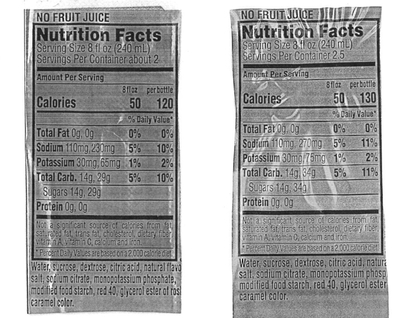# Calories in a sports drink

Alignments to Content Standards: N-Q.A.3

The label on a $16.9$ ounce bottle of a sports drink indicates that one serving of $8$ ounces contains $50$ calories.

1. Based on this information, about how many calories are in the full bottle?
2. The label also says that the full bottle contains $120$ calories. Does this agree with your estimate from part (a)? How can you explain the discrepancy (if there is a discrepancy)?
3. The label on a 20 ounce bottle of the same sports drink says the bottle contains $130$ calories. Is this consistent with the information on the $16.9$ ounce bottle?

## IM Commentary

This problem involves the meaning of numbers found on labels. When the level of accuracy is not given we need to make assumptions based on how the information is reported. An unexpected surprise awaits in this case, however, as no reasonable interpretation of the level of accuracy makes sense of the information reported on the bottles in parts (b) and (c). Either a miscalculation has been made or the numbers have been rounded in a very odd way.

The goal of the task is to stimulate a conversation about rounding and about how to record numbers with an appropriate level of accuracy, tying in directly to the standard N-Q.3. It is therefore better suited for instruction than for assessment purposes. The teacher may wish to bring in bottles with the indicated information and have students read the labels and think about what is recorded there before giving them this problem.

Students may wish to study in more detail government regulations regarding nutritional information on food labels. For this problem, the following website discusses how numbers are supposed to be rounded:

Food Labeling Guidelines

The numbers remain problematic after reading this documentation! Below is a picture of these labels:The label on the left is for the 16.9 ounce bottle and the label on the right for the 20 ounce bottle.

## Solutions

Solution: 1

1. Since there are $16.9$ ounces in the bottle and $8$ ounces per serving this means that there are $16.9 \div 8$ servings in the bottle. With $50$ calories per serving this measn that there are $$(16.9 \div 8) \times 50 \,\,\,\mbox{calories}$$ in the bottle. This is $105 \frac{5}{8}$ calories. Without knowing the level of accuracy of the reported $50$ calories per serving, it is not clear whether or not this number, $105 \frac{5}{8}$, should be rounded and, if so, to which digit.
2. The reported $120$ calories is substantially more (over ten percent) than the calculated $105 \frac{5}{8}$ from part (a). One explanation of this would be that the $50$ calories per serving is rounded to the nearest $10$. So there could just less than $55$ calories per $8$ ounce serving. To make a calculation, suppose that there care in fact $54.5$ calories in an $8$ ounce serving. Working as in part (a), this would mean that there are $$(16.9 \div 8) \times 54.5 \,\,\,\mbox{calories}$$ in the bottle. This is just a little more than $115$ calories and so, rounding to the nearest $10$, we would get the reported $120$ calories for the $16.9$ ounce bottle.
3. If we assume that all numbers are rounded to the nearest $10$ as in part (b) then in order for the $16.9$ ounce bottle to have $115$ calories (and therefore be rounded to the recorded $120$ calories), we would need each $8$ ounce serving to have at least $$\frac{115}{\frac{16.9}{8}} \approx 54.4 \,\,\,\mbox{calories}.$$ Now the $20$ ounce bottle has $2 \frac{1}{2}$ servings of $8$ ounces and so this would mean that it would have $$2 \frac{1}{2} \times 54.4 = 136 \,\,\mbox{calories}.$$ This is not possible since this would be rounded to $140$ rather than the reported $130$.

The numbers are close enough that it is possible a simple mathematical error has been made. It is difficult to find another plausible explanation since these numbers do not appear to be rounded to anything more coarse than to the nearest $10$.

Solution: 2 Alternate solution to part (c)

Suppose $x$ denotes the number of calories per ounce in the sports drink. If the number of calories in the $20$ ounce bottle, rounded to the nearest $10$, is $130$ then this means that $$125 \leq 20x \lt 135.$$ We can scale this inequality in order to get information about the number of calories in the $16.9$ ounce bottle. We can do this by multiplying all three terms in the inequality by $\frac{16.9}{20}$. This gives $$105.625 \leq 16.9x \lt 114.075.$$ This means that, rounded to the nearst $10$, the number of calories in the $16.9$ ounce bottle should be reported as $110$.

Similarly if $120$ calories is correct, to the nearest $10$, for the $16.9$ ounce bottle, this means that $$115 \leq 16.9x \lt 125.$$ Scaling this inequality by $\frac{20}{16.9}$ shows that the number of calories in the $20$ ounce bottle can only round to $140$ or $150$, not to the $130$ listed on the $16.9$ ounce bottle.